# stl中push_back和浅拷贝和深拷贝的问题

《程序员面试宝典》中stl模板与容器中的一个例子：

#include <iostream>
#include <cstdlib>
#include <vector>
#include <string.h>
#include <stdio.h>

using namespace std;

class CDemo{
public:
CDemo():str(NULL){};
~CDemo(){
if(str) delete[] str;
};

char * str;
};

int main(){
CDemo d1;
d1.str=new char;
strcpy(d1.str,"trend micro");

vector<CDemo> * a1=new vector<CDemo>;
a1->push_back(d1);
delete a1;

getchar();
printf("getchar");
return 0;
}~CDemo(){
static int i=0;
cout<<"&CDemo"<<i++<<"="<<(int *)this<<",str="<<(int *)str<<endl;
if(str) delete[] str;
};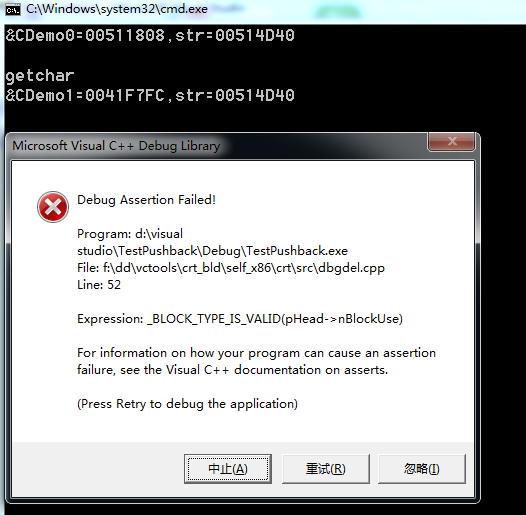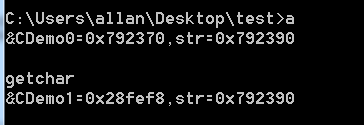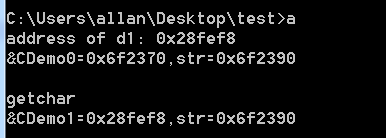#include <iostream>
#include <cstdlib>
#include <vector>
#include <string.h>
#include <stdio.h>

using namespace std;

class CDemo{
public:
CDemo():str(NULL){
cout<<"construct invoke!"<<endl;

};
CDemo(const CDemo &cd){
cout<<"copy construct invoke!"<<endl;
cout<<"address of copy:"<<(int *)this<<endl;
this->str=cd.str;
}
~CDemo(){
static int i=0;
cout<<"deconstruct &CDemo"<<i++<<"="<<(int *)this<<",str="<<(int *)str<<endl;
if(str) delete[] str;
};

char * str;
};

int main(){
CDemo d1;
cout<<"address of d1: "<<&d1<<endl;
d1.str=new char;
strcpy(d1.str,"trend micro");

vector<CDemo> * a1=new vector<CDemo>;
a1->push_back(d1);
delete a1;

return 0;
}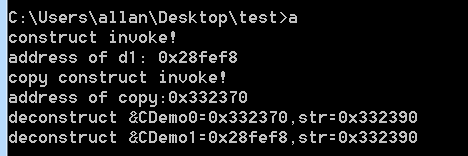CDemo(const CDemo &cd){
cout<<"copy construct invoke!"<<endl;
cout<<"address of copy:"<<(int *)this<<endl;
this->str=new char[strlen(cd.str)+1];
strcpy(str,cd.str);

}

STL 的 vector push_back 类对象时出现问题，偶尔发现 vector 在 push_back 时的调用类对象的拷贝构造函数和析构函数有点特别，简单做下分析。

#include <iostream>
#include <vector>

using namespace std;

struct sss
{
public:
explicit sss(int val) : value(val)
{
cout << "---init sss " << this << ", value:" << value << endl;
}

sss(const sss& org)
{
cout << "---copy " << &org << " to " << this << endl;
value = org.value;
}

~sss()
{
cout << "---destory sss " << this << ", value:" << value << endl;
}

int value;
};

int main(int argc, char ** argv)
{
sss s_tmp(11);
int i = 0;
vector<sss> vvv;

for (i = 0; i < 5; i++) {
s_tmp.value++;
vvv.push_back(s_tmp);
cout << "size: " << vvv.size() << ", capacity: " << vvv.capacity() << endl;
}

return 0;
}

g++中编译的结果如下图：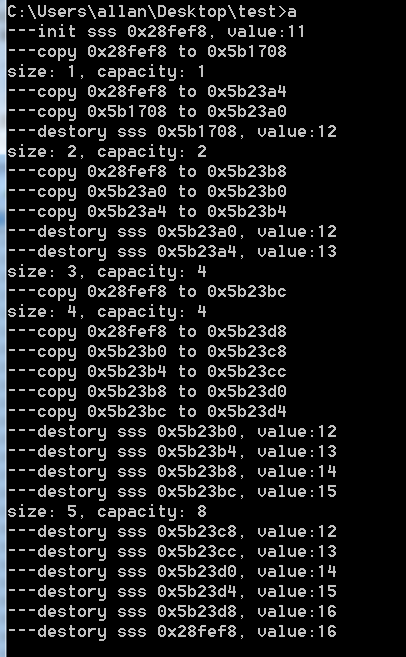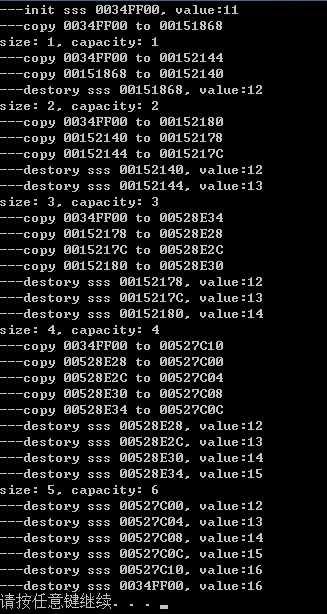vector 每次调用 push_back 时都会拷贝一个新的参数指定的 sss 类对象，这会调用 sss 的拷贝构造函数，第一次的 copy 正常，而且 vector 的实际容量也由 0  变为 1。

在Visual Studio 2008 中发现 vector 的实际空间增加顺序为：1 - 2 - 3 - 4 - 6 - 9 - 13 - 19 - 28 - 42 - 63 - 94 - 141 - 211 …

void push_back(const _Ty& _Val)

void push_back(const _Ty& _Val)
{
// insert element at end
if (size() < capacity())
#if _HAS_ITERATOR_DEBUGGING
{
// room at end, construct it there
_Orphan_range(_Mylast, _Mylast);
_Mylast = _Ufill(_Mylast, 1, _Val);
}
#else /* _HAS_ITERATOR_DEBUGGING */
_Mylast = _Ufill(_Mylast, 1, _Val);
#endif /* _HAS_ITERATOR_DEBUGGING */
else
insert(end(), _Val);
}